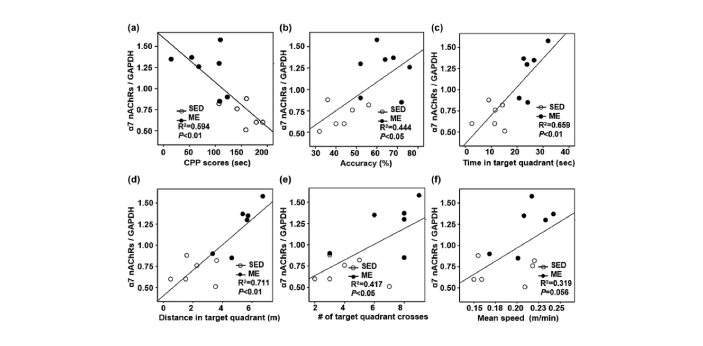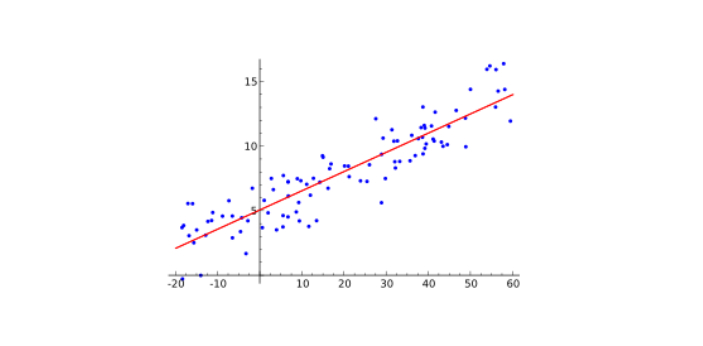What is the difference between Correlation and Regression? - ProProfs Discuss+ Ask Question

# What is the difference between Correlation and Regression?

Asked by Isa , Last updated: Mar 11, 2023

###Request 0FollowShareAnswer AnonymouslyAnswer LaterCopy LinkK. ShadrachWhen referring to correlation with respect to two or more variables, it simply denotes that the variables are related to themselves in one way or the other. Correlation analysis is employed to determine if there is a relationship between variables, and also check the strength of the relationship. If there is a relationship between two variables (independent) and b (dependent) such that the variation in the magnitude of the dependent variable accompanies the variation in magnitude of the independent variable, then you can say there is a correlation between the two variables. In addition, correlation can be classified, whether into a linear or nonlinear one.In contrast, regression analysis makes use of existing data. It checks the mathematical relationship between variables, and this can be used to calculate the value of the dependent variable by making reference to the value of the independent variable. Correlation chooses a random value for both dependent and independent variables, but regression does not need to use random variables.F. Hope

Regression is a statistical method used to connect the relation between the variables. Often when information is collected, there may be a variable that is dependent on others. Regression methods can only determine the exact relationship between those variables. The most common way regression is used to calculate the value of regression analysis to estimate the value of the contingent variable for a given value or range of values of the independent variable using regression. By using regression, the relationship is also established.On the other hand, correlation is a gauge of the intensity of the association between two variables. The parallel coefficient quantifies the degree of change in one variable based on the change in other variables. In statistics, correlation is linked to the concept of dependence, which is the statistical connection between two variables.Search for Google imagesSelect a recommended image
Upload from your computerCancelSearch for Google imagesSelect a recommended image
Upload from your computerCancelSearch for Google imagesSelect a recommended image
Upload from your computerCancel